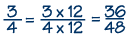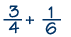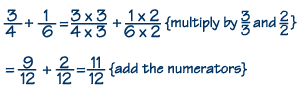Home    |    Teacher    |    Parents    |    Glossary    |    About UsIt's easy to add and subtract like fractions, or fractions with the same denominator. You just add or subtract the numerators and keep the same denominator. The tricky part comes when you add or subtract fractions that have different denominators. To do this, you need to know how to find the least common denominator. In an earlier lesson, you learned how to simplify, or reduce, a fraction by finding an equivalent, or equal, fraction where the numerator and denominator have no common factors. To do this, you divided the numerator and denominator by their greatest common factor.

In this lesson, you'll learn that you can also multiply the numerator and denominator by the same factor to make equivalent fractions.

Example 1In this example, since 12 divided by 12 equals one, and any number multiplied by 1 equals itself, we know 36/48 and 3/4 are equivalent fractions, or fractions that have the same value. In general, to make an equivalent fraction you can multiply or divide the numerator and denominator of the fraction by any non-zero number.

Since only like fractions can be added or subtracted, we first have to convert unlike fractions to equivalent like fractions. We want to find the smallest, or least, common denominator, because working with smaller numbers makes our calculations easier. The least common denominator, or LCD, of two fractions is the smallest number that can be divided by both denominators. There are two methods for finding the least common denominator of two fractions:

Example 2Method 1:
Write the multiples of both denominators until you find a common multiple.

The first method is to simply start writing all the multiples of both denominators, beginning with the numbers themselves. Here's an example of this method. Multiples of 4 are 4, 8, 12, 16, and so forth (because 1 × 4=4, 2 × 4=8, 3 × 4=12, 4 × 4=16, etc.). The multiples of 6 are 6, 12,…--that's the number we're looking for, 12, because it's the first one that appears in both lists of multiples. It's the least common multiple, which we'll use as our least common denominator.

Method 2:
Use prime factorization.

For the second method, we use prime factorization-that is, we write each denominator as a product of its prime factors. The prime factors of 4 are 2 times 2. The prime factors of 6 are 2 times 3. For our least common denominator, we must use every factor that appears in either number. We therefore need the factors 2 and 3, but we must use 2 twice, since it's used twice in the factorization for 4. We get the same answer for our least common denominator, 12.

Example 3

prime factorization of 4 = 2 × 2
prime factorization of 6 = 2 × 3
LCD = 2 × 2 × 3 = 12

Now that we have our least common denominator, we can make equivalent like fractions by multiplying the numerator and denominator of each fraction by the factor(s) needed. We multiply 3/4 by 3/3, since 3 times 4 is 12, and we multiply 1/6 by 2/2, since 2 times 6 is 12. This gives the equivalent like fractions 9/12 and 2/12. Now we can add the numerators, 9 + 2, to find the answer, 11/12.

Example 4Homework Help | Pre-Algebra | FractionsEmail this page to a friendSearch·  Definitions·  Reducing fractions·  Adding and subtract-     ing fractions·  Multiplying fractions·  Dividing fractions·  Adding and subtract-     ing mixed numbers·  Multiplying     mixed numbers·  Dividing     mixed numbersFirst Glance In Depth Examples WorkoutAdding and subtracting fractions